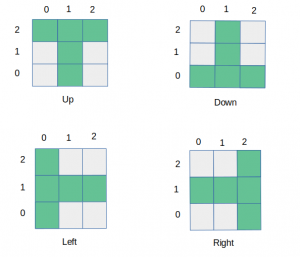# Check if the given 2-D points form T-shape or not

Given the coordinates of 5 2-dimensional points, check if they form a closed T shape. Print ‘Yes’ if they form T shape and ‘No’ otherwise. Note: Coordinates should be distinct and integers.

There are 4-types of T shaped formations possible according to the given conditions:Examples:

```Input: [[7, 5], [8, 5], [6, 5], [7, 7], [7, 6]]
Output: Yes

Input: [[0, 0], [1, 0], [2, 0], [1, -1], [1, -2]]
Output: Yes
```

## Recommended: Please try your approach on {IDE} first, before moving on to the solution.

Approach:

1. Consider the first point in the given list as the centre (x, y) (i.e intersection of the two lines that form T-shape).
2. Then check if all the points which are needed to form T-shape of which (x, y) is the centre are present in the list of given points or not.
3. Check this for all the 4 possible patterns of T-shape.
4. Repeat this process from step-1 for all points in the list until a solution is found.

Below is the implementation of the above approach:

 `# Python3 code to check if given 5  ` `# 2-D points form T-shape or not ` ` `  `# Import the function to initialize the ` `# dictionary with a specific value ` `from` `collections ``import` `defaultdict ` ` `  `# This function checks if the points ` `# form T-shape pointing up ` `def` `isUpDirected(point, x, y): ` `     `  `    ``return` `(point[(x``-``1``, y)] ``and` `            ``point[(x, y)] ``and` `            ``point[(x ``+` `1``, y)] ``and` `            ``point[(x, y``-``1``)] ``and` `            ``point[(x, y``-``2``)]) ` ` `  `# This function checks if the points ` `# form T-shape pointing down ` `def` `isDownDirected(point, x, y): ` `     `  `    ``return` `(point[(x``-``1``, y)] ``and` `            ``point[(x, y)] ``and` `            ``point[(x ``+` `1``, y)] ``and` `            ``point[(x, y ``+` `1``)] ``and` `            ``point[(x, y ``+` `2``)]) ` `             `  `# This function checks if the points ` `# form T-shape pointing left ` `def` `isLeftDirected(point, x, y): ` `     `  `    ``return` `(point[(x, y ``+` `1``)] ``and` `            ``point[(x, y)] ``and` `            ``point[(x, y``-``1``)] ``and` `            ``point[(x ``+` `1``, y)] ``and` `            ``point[(x ``+` `2``, y)]) ` ` `  `# This function checks if the points ` `# form T-shape pointing right ` `def` `isRightDirected(point, x, y): ` `     `  `    ``return` `(point[(x, y ``+` `1``)] ``and` `            ``point[(x, y)] ``and` `            ``point[(x, y``-``1``)] ``and` `            ``point[(x``-``1``, y)] ``and` `            ``point[(x``-``2``, y)]) ` ` `  ` `  `# This function checks if given points ` `# form a T-shape or not ` `def` `solve(grid): ` ` `  `    ``# Initialize the dictionary with False value ` `    ``point ``=` `defaultdict(``lambda` `: ``False``) ` `    ``flag ``=` `False` ` `  `    ``for` `i ``in` `range``(``len``(grid)): ` ` `  `        ``# Assign True value to the points which ` `        ``# are present in the given list ` `        ``point[(grid[i][``0``], grid[i][``1``])] ``=` `True` ` `  `    ``for` `i ``in` `range``(``len``(grid)): ` ` `  `        ``# Check if the given points form any of the ` `        ``# 4 possible T-shaped formations ` `        ``if` `isUpDirected(point, grid[i][``0``], grid[i][``1``]) \ ` `            ``or` `isDownDirected(point, grid[i][``0``], grid[i][``1``]) \ ` `            ``or` `isLeftDirected(point, grid[i][``0``], grid[i][``1``]) \ ` `            ``or` `isRightDirected(point, grid[i][``0``], grid[i][``1``]): ` ` `  `            ``flag ``=` `True` `            ``break` ` `  `    ``if` `flag ``=``=` `True``: ` `        ``return` `'Yes'` `    ``else``: ` `        ``return` `'No'` ` `  `print``(solve([[``7``, ``5``], [``8``, ``5``], [``6``, ``5``], [``7``, ``7``], [``7``, ``6``]])) ` `print``(solve([[``0``, ``0``], [``1``, ``0``], [``2``, ``0``], [``1``, ``-``1``], [``1``, ``-``2``]])) `

Output:

```Yes
Yes
```

Time Complexity: O(1)My Personal Notes arrow_drop_upI like solving puzzles

If you like GeeksforGeeks and would like to contribute, you can also write an article using contribute.geeksforgeeks.org or mail your article to contribute@geeksforgeeks.org. See your article appearing on the GeeksforGeeks main page and help other Geeks.

Please Improve this article if you find anything incorrect by clicking on the "Improve Article" button below.